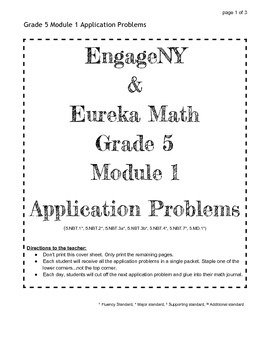# EngageNY Grade 5 Module 1 Application ProblemsSubject
Resource Type
File Type

PDF

(173 KB|3 pages)
Standards
• Product Description
• StandardsNEW
Engage NY and Eureka Math Grade 5 Module 1 Application Problems. These are all the Application Problems for Grade 5, Module 1. Print one packet per student. Students cut off the daily application problem and then glue it into their math journals.
Add, subtract, multiply, and divide decimals to hundredths, using concrete models or drawings and strategies based on place value, properties of operations, and/or the relationship between addition and subtraction; relate the strategy to a written method and explain the reasoning used.
Use place value understanding to round decimals to any place.
Compare two decimals to thousandths based on meanings of the digits in each place, using >, =, and < symbols to record the results of comparisons.
Read and write decimals to thousandths using base-ten numerals, number names, and expanded form, e.g., 347.392 = 3 × 100 + 4 × 10 + 7 × 1 + 3 × (1/10) + 9 × (1/100) + 2 × (1/1000).
Explain patterns in the number of zeros of the product when multiplying a number by powers of 10, and explain patterns in the placement of the decimal point when a decimal is multiplied or divided by a power of 10. Use whole-number exponents to denote powers of 10.
Total Pages
3 pages
Not Included
Teaching Duration
N/A
Report this Resource to TpT
Reported resources will be reviewed by our team. Report this resource to let us know if this resource violates TpT’s content guidelines.